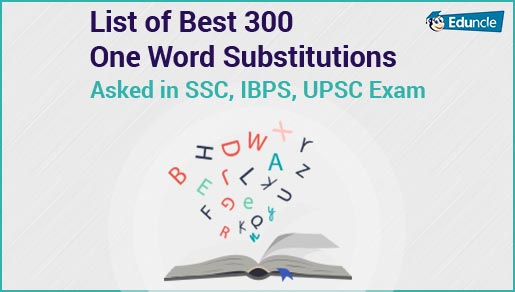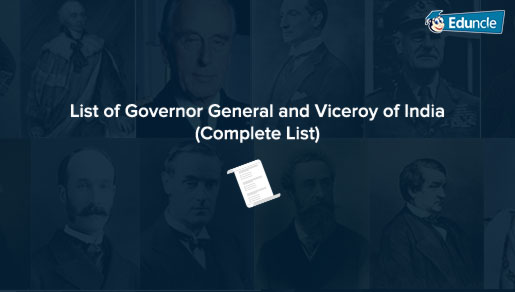Government Jobs FollowRavi @Eduncle posted an Article
June 22, 2019 • 12:32 pm

# How to Solve Average Questions – Know About Tips, Tricks and Concepts!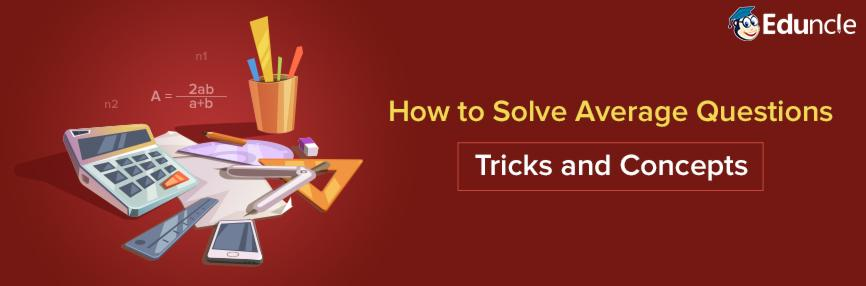As the level of numerical ability questions in competitive exams is getting complex every year, there are some topics which are still there as a helping hand.

Yes, we are talking about the questions asked from the topic ‘Average’.

This topic could be counted in the easiest topics of Numerical Ability Section.

Candidates who want to practice for the Average questions to boost up their score in the exam must read this article.

Here we have catered all the important Average Formulas and Tricks that can help you to qualify for the exam.

## What is Average? Know the Average Meaning Here!

As per the Average Definition, “A calculated central value of a set of numbers” is known as Average or we can say that the results that we get by adding several quantities and then dividing the total by the number of quantities is called Average.

There are various formulas that are used to calculate the complete Average Problems.

So, below in this blog, you will get all the terms related to solve the ‘Average Questions” that are usually asked in the exams like IBPS PO, IBPS Clerk, SSC and in other competitive exams.

Probability Aptitude

## Average Aptitude Questions – Know the Important Formulae!

There are some general formulas used to solve the different types of Average Questions. All these formulas are based on the numbers and conditions in an Average Question.

Below we have catered some useful formulas related to Average Problems that can help you to get a perfect solution in few steps.

 Average of first ‘n’ natural numbers (n+1)/2 Average of first even ‘n’ numbers (n+1) Average of first odd ‘n’ numbers N Average of 1 to ‘n’ odd numbers (Last odd number +1)/2 Average of 1 to ‘n’ even numbers (Last even number+2)/2 Average Squares of first ‘n’ natural numbers (n+1)(2n+1)/6 Average of Cubes of first ‘n’ natural numbers n2(n+1)2/4 Average of the Rest of the Observations ma-nb/m-n

The formulas that we have discussed in the above-mentioned list are used to solve all types of basic Average Problems.

But nowadays, Average Problems are likely to be asked on a different pattern.

Exams like IBPS PO and SBI PO covers the new type Average Speed Problems.

From the table given below, you can check the list of Formulae related to Average Speed.

Download Railway Exam study material and prepare for RRB NTPC, JE-SSE, ALP, Group C & D!

 If a person covers a certain distance at a speed of A km/h and again covers the same distance at a speed of B km/h, the Average Speed during the whole journey will be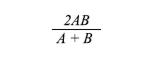If a person covers three equal distances at a speed of A km/h, B km/h and C km/h and C km/h, then the average speed during the whole journey will be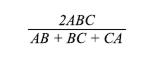If distance ‘P’ is covered with speed ‘X’, distance ‘Q’ is covered with speed ‘Y’ and ‘R’ is covered with speed ‘Z’, then the average speed will be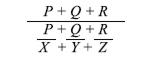If a person covers ‘P’ distance of his total journey with speed of ‘X’, Q distance of total journey with speed of ‘Y’ and ‘R’ distance of total journey with speed of ‘Z’ then the Average Speed will be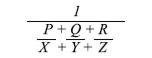For a better understanding you need and use of all these formulae, below we have shared an example that can help you to clear the concept that how to calculate average.

Example –

A man divides his total journey distance into three alike parts and then he travels each part of distance travel with the speeds of 20 km/hr, 15 km/h, and 10 km/hr respectively. During the whole journey what was is average speed?

Solution –

To solve this problem, we need to pick the formula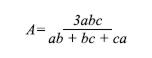Here, the values of a, b and c are represented by the speeds of the person for different parts.

So,

a = 20 km/hr

b = 15 km/hr

c = 10 km/hr

= 3×20×15×10/(20×15) + (15×10) + (20×10)After solving all the values, the final answer that we get is 180/13.

Here you have reached at the end of the blog. We hope that the above-mentioned formulas and example are helpful to understand the concepts of solving Average Questions.

To score well from this topic all you need to do is to learn the formulas. You can use a sticky note, flashcards, etc.

If you have any problem to understand the problem-solving concept of Average, then ask with our experts through the comments’ box below.

You Might Also Like